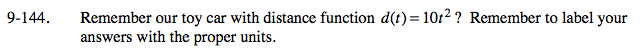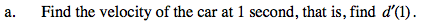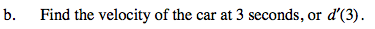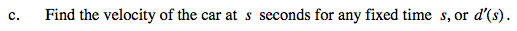### Home > PC > Chapter 9 > Lesson 9.3.4 > Problem9-144

9-144.
1. Remember our toy car with distance function d(t) = 10t2? Remember to label your answers with the proper units. Homework Help ✎

1. Find the velocity of the car at 1 second, that is, find d ′(1).

2. Find the velocity of the car at 3 seconds, or d ′(3).

3. Find the velocity of the car at s seconds for any fixed time s, or d ′(s).Find the slope function.

$d'(t)=\lim_{h\rightarrow 0}\frac{10(t+h)^{2}-10t^{2}}{h}$Substitute t = 1 into the slope function.Substitute t = 3 into the slope function.Substitute t = s into the slope function.